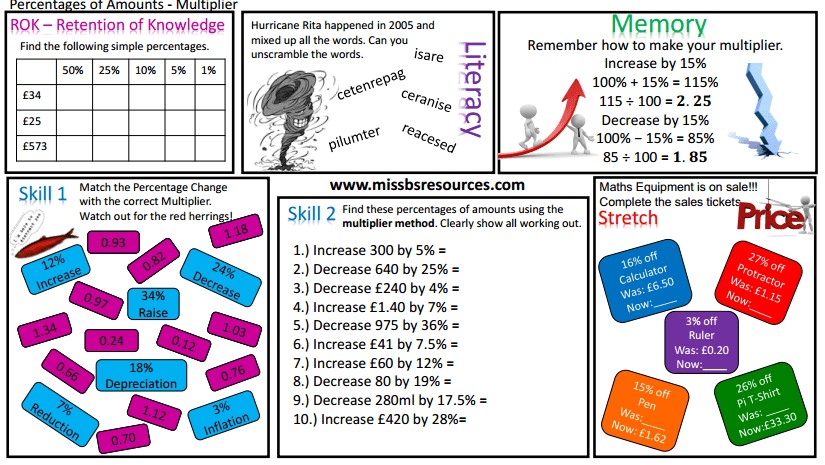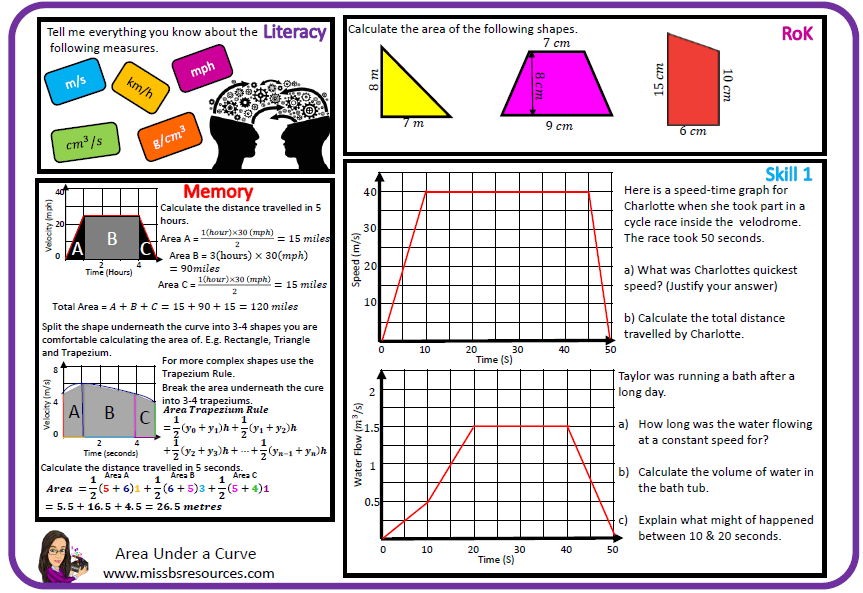0
Shares

# Number Skill Review

These maths resources include bronze, silver and gold maths tasks for the end of a module or revision. They are ideal for use for students who are in their maths GCSE years, as they are based around exam style maths questions. These resources are to be used as an aid to review maths skills, see how far you can get within an appropriate time limit. (10 - 20 minutes usually). These maths resources should challenge and stretch students on a topic as either pre or post assessment.

The newer skill reviews I have been making try to focus in on the separate AO1 (Calculate), AO2 (Reasoning) and AO3 (Problem Solving) maths Skills. Last year I focused on creating skill review maths worksheets on the new maths GCSE Topics, such as error intervals and truncation. Most maths skill review sheets have answers already provided. However some of the older maths resources don't. If you have a completed set of answers for any of the older maths skill reviews and are happy for me to share them please email them to me.

## Number Homework

@DIRT_expert recently introduced to PRET style homework's. PRET stands for Practise, Recall, Extend and Think. The homework's are designed to challenge students as well as support them with knowledge gained from lessons. My versions can be seen below, if I don't have what you need more can be found at PretHomework. These PRET homework's are great for getting students to think about their maths problem solving (AO3) and reasoning skills (AO2) as well exploring their love of maths more through research. It gives students a chance to excel and shine.

## Number Worksheets

I have developed my own style of differentiated maths worksheets for lessons which are inspired by the PRET Homework’s. These maths worksheets on algebra are split into several sections. Sometimes it will be relevant for these maths resources to be used over multiple lessons.

Memory – The memory boxes are there to provide students with memory aids or examples. The memory box does not mean necessarily this is the way to start teaching a topic. The maths memory tools support the great teaching within a lesson. Feel free to ignore this box also.

Literacy – Each maths worksheet includes a literacy section. Here students will be asked to recognise key maths words and define them. Alternatively students may be asked to describe a mathematical process. There has been a lot of research carried out which suggests those students who are able to talk about and explain the maths processes often understand and remember larger proportions of the work.

ROK – This stands for Retention of Knowledge. This is a chance for a student to recall their prior maths learning. The maths skills questioned in the retention section give students a chance to practise maths skills they will need to access the lesson. This helps build in a little bit of spaced learning.

Skills – The maths skill sections of the worksheet focus in on the developing new concepts taught on a maths topic. The maths questions often increase in difficulty throughout a skill section. These focus on the maths GCSE AO1 calculation skills.

Stretch – These are maths questions designed to stretch and challenge a student. These challenging maths questions interleave topics from other mathematical topics. Stretch questions focus on a student’s ability to mathematically reason (AO2) and problem solve (AO3). These allow students to explore the breadth and depth of a maths topic.Multipliers - Percentage Increase and DecreaseArea Underneath a Curve (Trapezium Rule)

### Danielle Bartram

#### Maths Lead Practitioner

Acklam Grange School
Middlesbrough
0
Shares